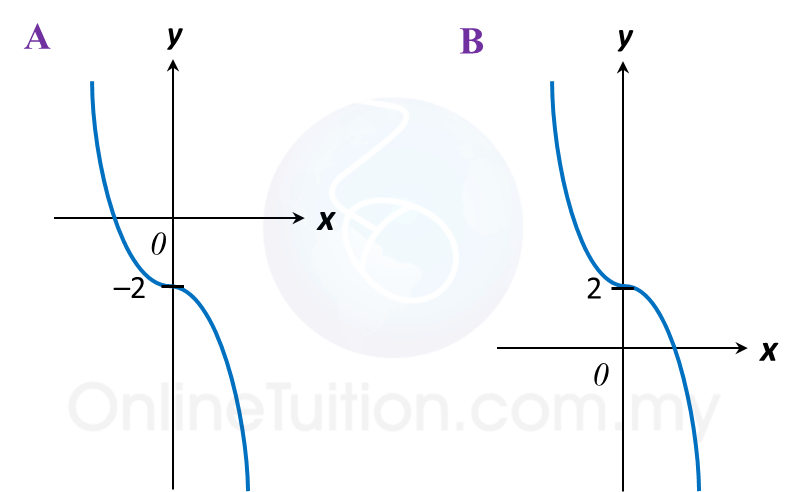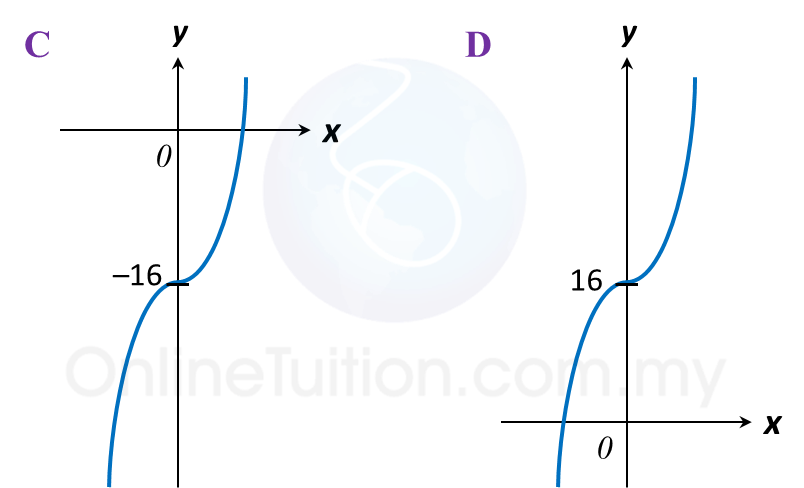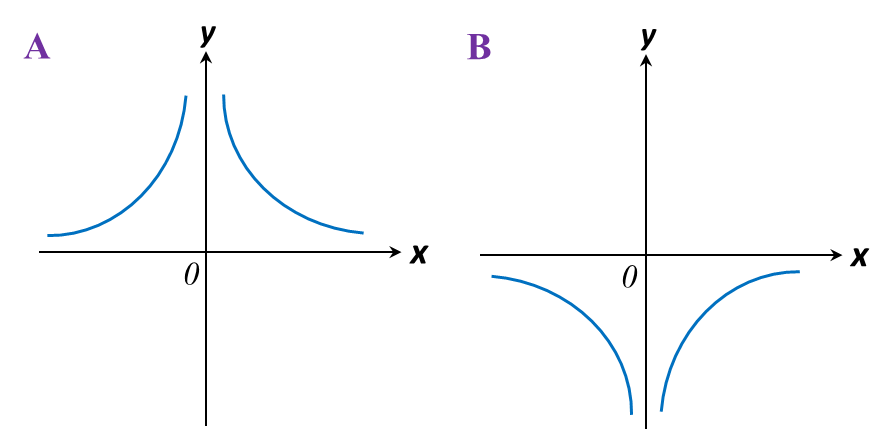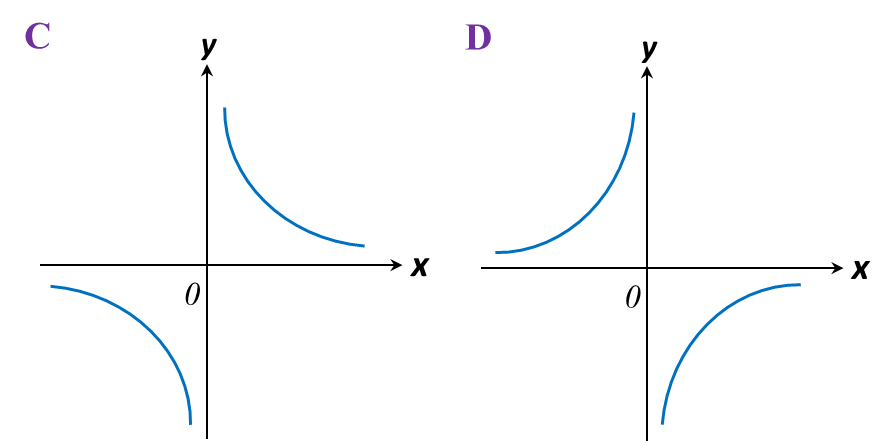# 2.4.2 Graph of Functions (II), SPM Paper 1 (Short Questions)

2.4.2 SPM Practice (Short Questions)

Question 3:
Which of the following graphs represent y = 2x– 16?Solution:
y = 2x3 – 16
On the y-axis, x = 0.
y = 2(0)3 – 16
y = –16

Question 4:
Which of the following graphs represent $y=-\frac{3}{x}?$Solution:
Highest power of the variable x is –1.
It is a reciprocal function